### MODEL Statement

MODEL dependent = regressors < / options > ;

The MODEL statement specifies the dependent variable and independent regressor variables for the regression model. If no independent variables are specified in the MODEL statement, only the mean (intercept) is estimated. To model a system of equations, specify more than one MODEL statement.

The following options can be used in the MODEL statement after a slash (/).

ESUPPORTS=( support (prior) … )

specifies the support points and prior weights on the residuals for the specified equation. The default is the following five support values: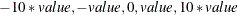where value is computed as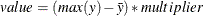for GME, where
is the dependent variable, and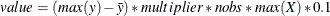for generalized maximum entropy—moments (GME-M), whereis the information matrix, and nobs is the number of observations. The multiplier depends on the MULTIPLIER= option. The MULTIPLIER= option defaults to 2 for unrestricted models and to 4 for restricted models. The prior probabilities default to the following: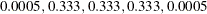The support points and prior weights are selected so that hypothesis tests can be performed without adding significant bias to the estimation. These prior probability values are ad hoc.

NOINT

suppresses the intercept parameter.

MARGINALS = ( variable = value, …, variable = value)

requests that the marginal effects of each variable be calculated for GME-D. Specifying the MARGINALS option with an optional list of values calculates the marginals at that vector of values. For example, if x1x4 are explanatory variables, then including

MARGINALS = ( x1 = 2, x2 = 4, x3 = –1, x4 = 5)

calculates the marginal effects at that vector. A skipped variable implies that its mean value is to be used.

CENSORED ( ( UB | LB) = (variable | value ), ESUPPORTS =( support (prior) …) )

specifies that the dependent variable be observed with censoring and specifies the censoring thresholds and the supports of the censored observations.

CATEGORY= variable

specifies the variable that keeps track of the categories the dependent variable is in when there is range censoring. When the actual value is observed, this variable should be set to MISSING.

RANGE ( ID = (QS | INT) L = ( NUMBER ) R = ( NUMBER ) , ESUPPORTS=( support <(prior)> … ) )

specifies that the dependent variable be range bound. The RANGE option defines the range and the key ( RANGE ) that is used to identify the observation as being range bound. The RANGE = value should be some value in the CATEGORY= variable. The L and R define, respectively, the left endpoint of the range and the right endpoint of the range. ESUPPORTS sets the error supports on the variable.